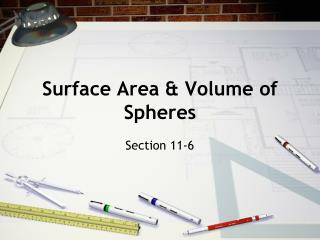DownloadDownload PresentationSurface Area & Volume of Spheres

# Surface Area & Volume of Spheres

Télécharger la présentation## Surface Area & Volume of Spheres

- - - - - - - - - - - - - - - - - - - - - - - - - - - E N D - - - - - - - - - - - - - - - - - - - - - - - - - - -
##### Presentation Transcript

1. Surface Area & Volume of Spheres Section 11-6

2. Vocab • Sphere - the set of all points in space equidistant from a given point called the center. • Radius - segment that has one endpoint at the center and the other endpoint on the sphere. • Diameter - segment passing through the center with endpoints on the sphere.

3. Surface Area of a Sphere

4. S.A. = 4 r 2Use the formula for the surface area of a sphere. 18 2 = 4 • 92Substitute r== 9. = 324 Simplify. The surface area of the sphere is 324 ft2. Find the surface area of the sphere below. Leave your answer in terms of .

5. Your turn • Find the S.A. of a sphere with a diameter of 14 in. Give your answer two ways, in terms of π and rounded to the nearest square inch. • 196π in2; 616 in2

6. Step 1: Use the circumference to find the radius in terms of . C=2 r Use the formula for circumference. 13 2 13=2 r Substitute 13 for C. 13 2 = r Solve for r. S.A. = 4r 2Use the formula for the surface area of a sphere. = 4• ( )2Substitute for r. 13 2 169 =Simplify. 53.794371Use a calculator. The circumference of a rubber ball is 13 cm. Calculate its surface area to the nearest whole number. Step 2: Use the radius to find the surface area. To the nearest whole number, the surface area of the rubber ball is 54 cm2.

7. Your turn • Find the S.A. of a melon with a circumference of 18 in. Round your answer to the nearest ten square inches. • 100 in2

8. Volume of a sphere

9. 4 3 V= r 3Use the formula for the volume of a sphere. 30 2 4 3 = • 153Substitute r = = 15. = 4500Simplify. The volume of the sphere is 4500 cm3. Find the volume of the sphere. Leave your answer in terms of .

10. Your turn • Find the volume to the nearest cubic inch of a sphere with a diameter of 60 inches. • 113,097 in3

11. 4 3 V= r 3Use the formula for the volume of a sphere. 4 3 1= r 3Substitute. 3 3 4 3 4 = r 3Solve for r 3. = r Find the cube root of each side. r0.62035049Use a calculator. The volume of a sphere is 1 in.3. Find its surface area to the nearest tenth. Step 1: Use the volume to find the radius r.

12. S.A. = 4r 2Use the formula for the surface area of a sphere. 4.8359744Use a calculator. 4(0.62035049)2Substitute. (continued) Step 2: Use the radius to find the surface area. To the nearest tenth, the surface area of the sphere is 4.8 in.2.

13. Your Turn • The volume of a sphere is 4200 ft3. Find S.A. to the nearest tenth. • 1258.9 ft3